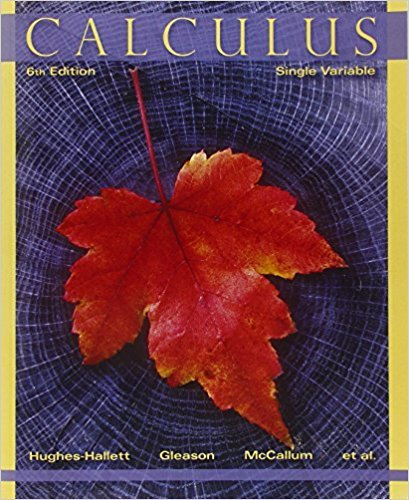×
×

# Solutions for Chapter Chapter 10: APPROXIMATING FUNCTIONS USING SERIES## Full solutions for Calculus: Single Variable | 6th Edition

ISBN: 9780470888643Solutions for Chapter Chapter 10: APPROXIMATING FUNCTIONS USING SERIES

Solutions for Chapter Chapter 10
4 5 0 306 Reviews
15
3
##### ISBN: 9780470888643

Since 64 problems in chapter Chapter 10: APPROXIMATING FUNCTIONS USING SERIES have been answered, more than 32451 students have viewed full step-by-step solutions from this chapter. Chapter Chapter 10: APPROXIMATING FUNCTIONS USING SERIES includes 64 full step-by-step solutions. Calculus: Single Variable was written by and is associated to the ISBN: 9780470888643. This expansive textbook survival guide covers the following chapters and their solutions. This textbook survival guide was created for the textbook: Calculus: Single Variable , edition: 6.

Key Calculus Terms and definitions covered in this textbook
• Annuity

A sequence of equal periodic payments.

• Arcsecant function

See Inverse secant function.

• Categorical variable

In statistics, a nonnumerical variable such as gender or hair color. Numerical variables like zip codes, in which the numbers have no quantitative significance, are also considered to be categorical.

• Direct variation

See Power function.

• First quartile

See Quartile.

• Line of symmetry

A line over which a graph is the mirror image of itself

• Linear function

A function that can be written in the form ƒ(x) = mx + b, where and b are real numbers

• Logarithmic regression

See Natural logarithmic regression

• Phase shift

See Sinusoid.

A procedure for fitting a quadratic function to a set of data.

• Range (in statistics)

The difference between the greatest and least values in a data set.

• Real number line

A horizontal line that represents the set of real numbers.

• Reciprocal identity

An identity that equates a trigonometric function with the reciprocal of another trigonometricfunction.

• RRAM

A Riemann sum approximation of the area under a curve ƒ(x) from x = a to x = b using x1 as the right-hand end point of each subinterval.

• Sample space

Set of all possible outcomes of an experiment.

• Statute mile

5280 feet.

• Sum of a finite geometric series

Sn = a111 - r n 2 1 - r

• Sum of an infinite series

See Convergence of a series

• Upper bound for ƒ

Any number B for which ƒ(x) ? B for all x in the domain of ƒ.

• Zoom out

A procedure of a graphing utility used to view more of the coordinate plane (used, for example, to find theend behavior of a function).

×